# Mechanical Engineering - Thermodynamics

### Exercise :: Thermodynamics - Section 6

21.

The value of one bar (in S. I. units) is equal to

 A. 1 x 102 N/m2 B. 1 x 103 N/m2 C. 1 x 104 N/m2 D. 1 x 105 N/m2

Explanation:

No answer description available for this question. Let us discuss.

22.

Petrol is distilled at

 A. 65° to 220°C B. 220° to 345°C C. 345° to 470°C D. 470° to 550°C

Explanation:

No answer description available for this question. Let us discuss.

23.

If the carbon content in the bituminous coal is 78 to 81%, it is said to be coking bituminous coal.

 A. Agree B. Disagree

Explanation:

No answer description available for this question. Let us discuss.

24.

The efficiency of the Carnot cycle is (where T1 and T2 = Highest and lowest temperature during the cycle)

 A.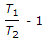B.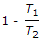C.D.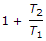Explanation:

No answer description available for this question. Let us discuss.

25.

The mass of excess air supplied is equal to

 A.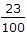x Mass of excess carbon B.x Mass of excess oxygen C.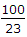x Mass of excess carbon D.x Mass of excess oxygen